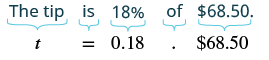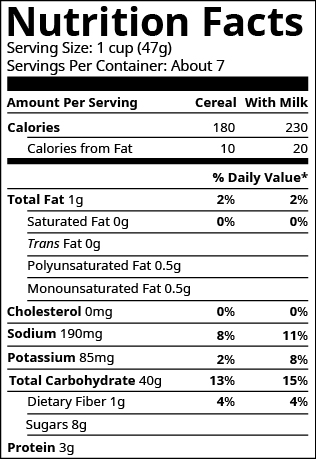Solving Applications With Percent

Learning Outcomes

• Calculate the tip for a meal given a percent
• Find total recommended amount of fiber and sodium given percent and amount
• Calculate percent calories from fat given total calories and calories from fat

Many applications of percent occur in our daily lives, such as tips, sales tax, discount, and interest. To solve these applications we’ll translate to a basic percent equation, just like those we solved in the previous examples in this section. Once you translate the sentence into a percent equation, you know how to solve it.

We will update the strategy we used in our earlier applications to include equations now. Notice that we will translate a sentence into an equation.

Solve an application

1. Identify what you are asked to find and choose a variable to represent it.
2. Write a sentence that gives the information to find it.
3. Translate the sentence into an equation.
4. Solve the equation using good algebra techniques.
5. Check the answer in the problem and make sure it makes sense.
6. Write a complete sentence that answers the question.

Now that we have the strategy to refer to, and have practiced solving basic percent equations, we are ready to solve percent applications. Be sure to ask yourself if your final answer makes sense—since many of the applications we’ll solve involve everyday situations, you can rely on your own experience.

example

Dezohn and his girlfriend enjoyed a dinner at a restaurant, and the bill was $\text{\68.50}$. They want to leave an $\text{18%}$ tip. If the tip will be $\text{18%}$ of the total bill, how much should the tip be?

Solution

 What are you asked to find? the amount of the tip Choose a variable to represent it. Let $t=$ amount of tip. Write a sentence that give the information to find it. The tip is 18% of the total bill. Translate the sentence into an equation.Multiply. $t=12.33$ Check. Is this answer reasonable? If we approximate the bill to $\text{\70}$ and the percent to $20\text{%}$, we would have a tip of $\text{\14}$. So a tip of $\text{\12.33}$ seems reasonable. Write a complete sentence that answers the question. The couple should leave a tip of $\text{\12.33}$.

try it

In the next video we show another example of finding how much tip to give based on percent.

example

The label on Masao’s breakfast cereal said that one serving of cereal provides $85$ milligrams (mg) of potassium, which is $\text{2%}$ of the recommended daily amount. What is the total recommended daily amount of potassium?example

Mitzi received some gourmet brownies as a gift. The wrapper said each brownie was $480$ calories, and had $240$ calories of fat. What percent of the total calories in each brownie comes from fat?

try it

The following video show another example of how to solve an application that involves percent.